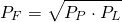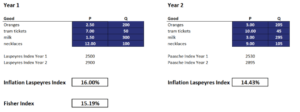# Fisher Index

The Fisher Index or Fisher Ideal Index is a consumer price index that combines the Laspeyres index and the Paasche index. The Fisher index was proposed by US economist Irving Fisher in the early 1930s. On this page we discuss Fisher ideal price index, explain why the fisher index is called ‘ideal’, and provide an implementation using an Excel spreadsheet.

## Fisher Index formula

The Fisher index formula is very simple, and uses as inputs the Laspeyres index and the Paasche index. In particular, the Fisher index is calculated as the geometric mean of the Laspeyres and the Paasche indexwhere Pp is the Paasche index and Pl is the Laspeyres index. Thus, application of the Fisher price index formula requires that we first calculate the Paasche and the Laspeyres index. Fortunately, both these indices are also straightforward to calculate using an Excel spreadsheet.

## Why is the Fisher index called ideal?

First, let’s briefly focus on why the Fisher index is called ideal. The reason the Fisher index is called the ideal index is twofold. Because it combines the Paasche index and the Laspeyres index, the index satisfies the time reversal test and the factor reversal test.

The time reversal test means that, if we change the base and current year in the formula, the product of the newly created index with the original index equals one. The factor reversal test means that if multiply the price index with a volume index of the same type, we get the change in the current values

The factor reversal test requires that when we multiply a price index and a volume index of the same type, the result should be equal to the proportionate change in the current values Thus, the Fisher index is an ideal index because it satisfies the time reversal test and the factor reversal test. This formula is used in the case when prices and quantities at the base and the observation period are quite different.

Using an Excel spreadsheet, we implement a Fisher index example starting from a set of prices and quantities. We saw earlier that this requires that we first implement the Paasche and the Laspeyres index. All the required computations are already implemented in the Excel spreadsheet. This illustrates that a simple Fisher index calculator can easily be implemented. The following figure illustrates the calculations## Summary

We discussed the Fisher index number in statistics. The index is often used to measure the consumer price index, which in turn is used to measure the inflation rate. Theoretically, the FI is the most robust way of calculating inflation because it is unbiased. In practice, however, most price indices are Laspeyres indices.

### Fisher Index calculator

Want to calculate the index using Excel? Download the Excel file: Fisher Index